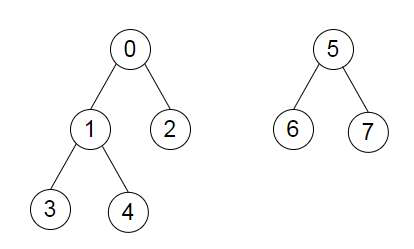# Program to Connect a Forest in Python

Suppose we have graphs as an adjacency lists. This graph is actually a set of disjoint trees. We have to add a certain number of edges to the forest such that it becomes a single tree. We have to return the minimum distance possible of the longest path between any two nodes. So, if the input is likethen the output will be 4.

We can add the edge 0 −> 5. Then, the longest path can be any of 3 −> 1 −> 0 −> 5 −> 7 or 4 −> 1 −> 0 −> 5 −> 7; and also these paths with the direction inverted. So we return the distance 4.

To solve this, we will follow these steps −

• seen := a new set

• dic := graph

• Define a function treeDepth(). This will take node.

• ret := 0

• Define a function dfs1(). This will take node, parent.

• add a node in set seen

• best2 := an empty min heap structure

• for each nxt in dic[node], do

• if nxt is not same as parent, then

• push(dfs1(nxt, node) + 1) into best2

• if len(best2) > 2, then

• pop from heap(best2)

• if best2 is empty, then

• return 0

• ret := maximum of ret, sum of all elements of best2

• return maximum of best2

• dfs1(node, null)

• return ret

• From the main method do the following −

• ret := 0, opt := a new list, sing := 0

• for node in range 0 to size of graph, do

• if node is present in seen, then

• go for next iteration

• res := treeDepth(node)

• sing := maximum of sing, res

• insert the ceiling of (res / 2) at the end of opt

• if size of opt <= 1, then

• return sing

• mx := maximum of opt

• for i in range 0 to size of opt, do

• if opt[i] is same as mx, then

• opt[i] := opt[i] − 1

• come out from the loop

• for i in range 0 to size of opt, do

• opt[i] := opt[i] + 1

• high2 := largest 2 elements from opt.

• return maximum of sum(high2) and sing

Let us see the following implementation to get better understanding −

## Example

Live Demo

import heapq, math
class Solution:
def solve(self, graph):
seen = set()
dic = graph
def treeDepth(node):
self.ret = 0
def dfs1(node, parent):
best2 = []
for nxt in dic[node]:
if nxt != parent:
heapq.heappush(best2, dfs1(nxt, node) + 1)
if len(best2) > 2:
heapq.heappop(best2)
if not best2:
return 0
self.ret = max(self.ret, sum(best2))
return max(best2)
dfs1(node, None)
return self.ret
ret = 0
opt = []
sing = 0
for node in range(len(graph)):
if node in seen:
continue
res = treeDepth(node)
sing = max(sing, res)
opt.append(int(math.ceil(res / 2)))
if len(opt) <= 1:
return sing
mx = max(opt)
for i in range(len(opt)):
if opt[i] == mx:
opt[i] −= 1
break
for i in range(len(opt)):
opt[i] += 1
high2 = heapq.nlargest(2, opt)
return max(sum(high2), sing)
ob = Solution()
graph = [
[1, 2],
[0,3,4],
,
,
,
[6,7],
,

]
print(ob.solve(graph))

## Input

graph = [
[1, 2],
[0,3,4],
,
,
,
[6,7],
,

]

## Output

4